# 14-240/Tutorial-December 2

## Boris

#### Theorem

Let$A$ be a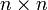$n \times n$ matrix and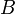$B$ be the matrix$A$ with two rows interchanged. Then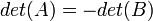$det(A) = -det(B)$. Boris decided to prove the following lemma first:

##### Lemma 1

Let$A$ be a$n \times n$ matrix and$B$ be the matrix$A$ with two adjacent rows interchanged. Then$det(A) = -det(B)$.

All we need to show is that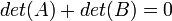$det(A) + det(B) = 0$. Assume that$B$ is the matrix$A$ with rows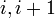$i, i + 1$ of$A$ interchanged. Since the determinant of a matrix with two identical rows is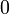$0$, then: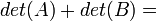$det(A) + det(B) =$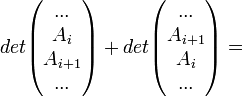$det\begin{pmatrix}...\\A_i\\A_{i + 1}\\...\end{pmatrix} + det\begin{pmatrix}...\\A_{i + 1}\\A_i\\...\end{pmatrix} =$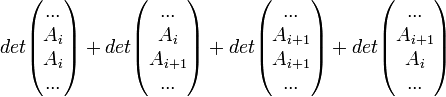$det\begin{pmatrix}...\\A_i\\A_i\\...\end{pmatrix} + det\begin{pmatrix}...\\A_i\\A_{i + 1}\\...\end{pmatrix} + det\begin{pmatrix}...\\A_{i + 1}\\A_{i + 1}\\...\end{pmatrix} + det\begin{pmatrix}...\\A_{i + 1}\\A_i\\...\end{pmatrix}$.

Since the determinant is linear in each row, then we continue where we left off:$det(A) + det(B) =$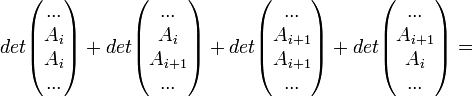$det\begin{pmatrix}...\\A_i\\A_i\\...\end{pmatrix} + det\begin{pmatrix}...\\A_i\\A_{i + 1}\\...\end{pmatrix} + det\begin{pmatrix}...\\A_{i + 1}\\A_{i + 1}\\...\end{pmatrix} + det\begin{pmatrix}...\\A_{i + 1}\\A_i\\...\end{pmatrix} =$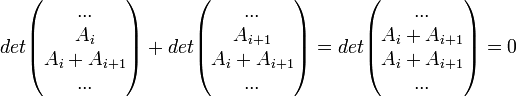$det\begin{pmatrix}...\\A_i\\A_i + A_{i + 1}\\...\end{pmatrix} + det\begin{pmatrix}...\\A_{i + 1}\\A_i + A_{i + 1}\\...\end{pmatrix} = det\begin{pmatrix}...\\A_i + A_{i + 1}\\A_i + A_{i + 1}\\...\end{pmatrix} = 0$.

Then$det(A) + det(B) = 0$ and$det(A) = -det(B)$. The proof of the lemma is complete.

For the proof of the theorem, assume that$B$ is the matrix$A$ with rows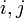$i, j$ of$A$ interchanged and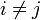$i \neq j$. By Lemma 1, we have the following: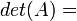$det(A) =$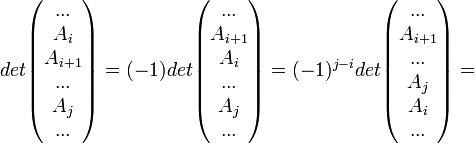$det\begin{pmatrix}...\\A_i\\A_{i + 1}\\...\\A_j\\...\end{pmatrix} = (-1)det\begin{pmatrix}...\\A_{i + 1}\\A_i\\...\\A_j\\...\end{pmatrix} = (-1)^{j - i}det\begin{pmatrix}...\\A_{i + 1}\\...\\A_j\\A_i\\...\end{pmatrix} =$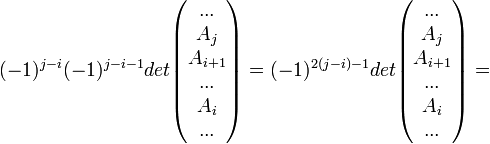$(-1)^{j - i}(-1)^{j - i - 1}det\begin{pmatrix}...\\A_j\\A_{i + 1}\\...\\A_i\\...\end{pmatrix} = (-1)^{2(j - i) - 1}det\begin{pmatrix}...\\A_j\\A_{i + 1}\\...\\A_i\\...\end{pmatrix} =$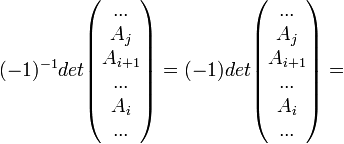$(-1)^{- 1}det\begin{pmatrix}...\\A_j\\A_{i + 1}\\...\\A_i\\...\end{pmatrix} = (-1)det\begin{pmatrix}...\\A_j\\A_{i + 1}\\...\\A_i\\...\end{pmatrix} =$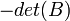$-det(B)$.

Then the proof of the theorem is complete.